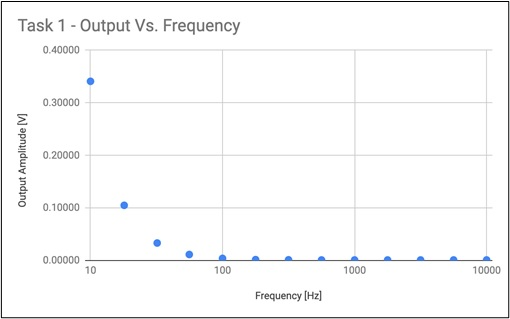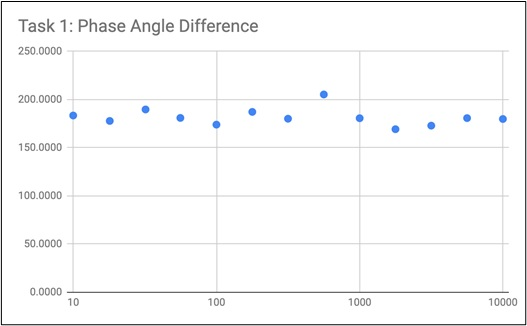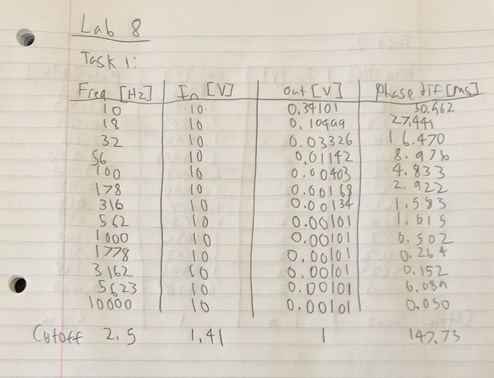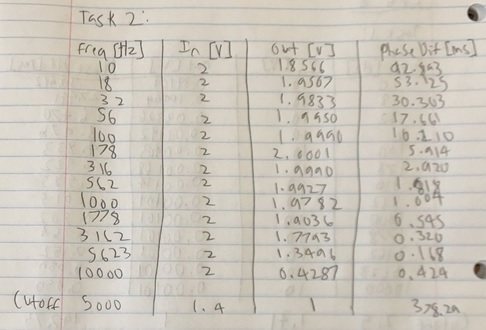# ECEN 214-516 Sinusoidal Steady State Response of a 2nd Order Circuit

ECEN 214-516

Post-Lab 8

Sinusoidal Steady State Response of a 2nd Order Circuit

Procedure

1. First we built the Sallen-Key circuit as shown in Figure 1.1. We used the values found in prelab for the values of R1, R2, C1,and C2.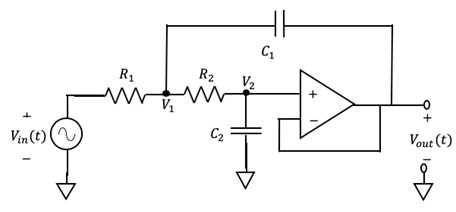Figure 1.1: Sallen-Key 2nd Order Circuit

1. We set up test probes so we would be able to test the input voltage, output voltage, and the phase difference between the input and output.
2. Next, we made those three measurements for thirteen different input frequencies.
3. Then, we changed the frequency to result of an input:output ratio of about 0.707.
4. Once the cut-off frequency was determined, the phase difference was also recorded.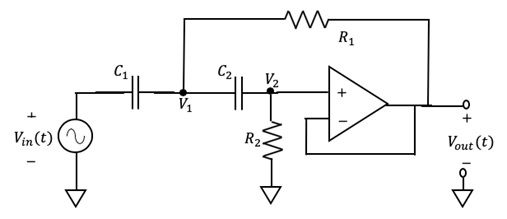1. First we built the Sallen-Key circuit as shown in Figure 2.1. We used the values found in prelab for the values of R1, R2, C1,and C2.

Figure 2.1: Modified Sallen-Key 2nd Order Circuit

1. We set up test probes so we would be able to test the input voltage, output voltage, and the phase difference between the input and output.
2. Next, we made those three measurements for thirteen different input frequencies.
3. Then, we changed the frequency to result of an input:output ratio of about 0.707.
4. Once the cut-off frequency was determined, the phase difference was also recorded.

Data and Results:

 Task 1 Frequency [Hz] Input Amplitude [V] Output Amplitude [V] Phase Diff [ms] 10 10 0.34101 50.962 18 10 0.10499 27.441 32 10 0.03326 16.470 56 10 0.01142 8.976 100 10 0.00403 4.833 178 10 0.00168 2.922 316 10 0.00134 1.583 562 10 0.00101 1.015 1000 10 0.00101 0.502 1778 10 0.00101 0.264 3162 10 0.00101 0.152 5623 10 0.00101 0.089 10000 10 0.00101 0.050 Cut-Off 2.5 1.41 1 147.73